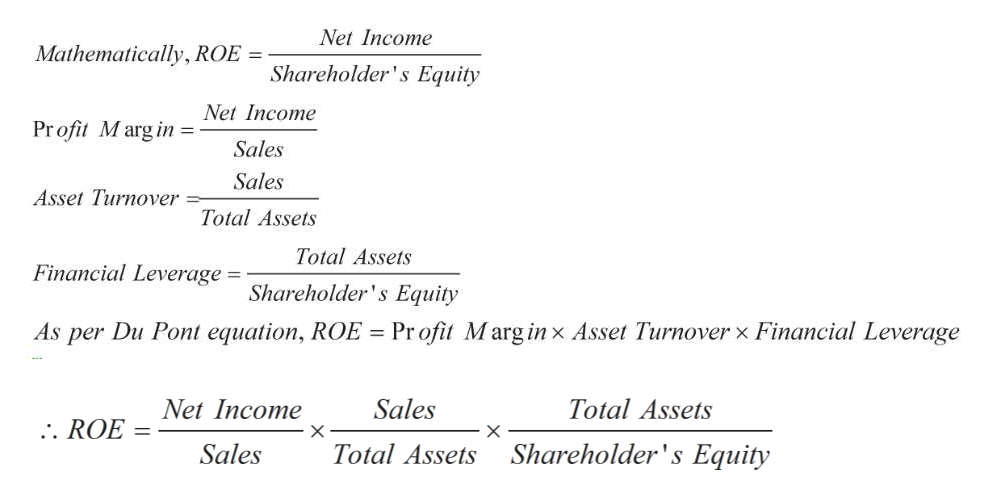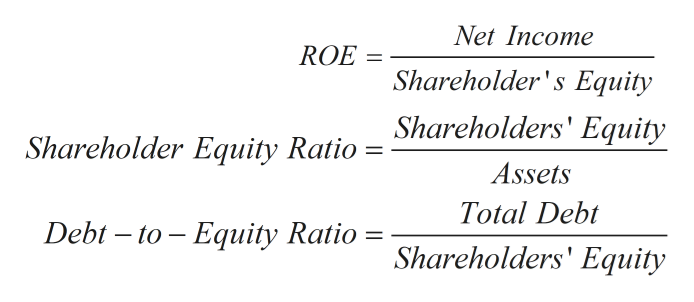# There are vairous measures to evaluate a company's finances.  What are three measures that look at shareholder equity as part of the formula?

Question
1 views

There are vairous measures to evaluate a company's finances.  What are three measures that look at shareholder equity as part of the formula?

check_circle

Step 1

Your question isn't very clear and could be answered in atleast two different ways. I will provide two solutions and I'm sure you'll know which one answers your question aptly.

Solution with Du Pont Equation:

Du Pont equation represents Return on Equity as product of three measures namely profit margin, asset turnover, and financial leverage.

Return on Equity is a measure of profitability of business and it indicates how efficiently income is generated with investments received from shareholders. Profit margin is a measure of profits as percentage of revenues/sales. Asset turnover ratio represents assets ability to generate sales. Financial leverage shows how much debt is used to acquire more assets.

Du Pont equation can be written as shown in the last line  below.help_outlineImage TranscriptioncloseNet Income Mathematically, ROE = Shareholder's Equity Net Income Pr ofit Marg in Sales Sales Asset Turnover Total Assets Total Assets Financial Leverage Shareholder's Equity Pr ofit Marg in x Asset Turnover x Financial Leverage As per Du Pont equation, ROE = Sales Total Assets Net Income .. ROE X - X = Shareholder's Equity Total Assets Sales fullscreen
Step 2

Solution with financial ratios:

Another interpretation of your question could be that you were looking for financial ratios which are calculated using shareholder's equity.

Three such important ratios are ROE, Shareholder equity ratio and Debt to Equity ratio.

ROE is a measure of profitability and shows what returns ae generated ...help_outlineImage TranscriptioncloseNet Income ROE = Shareholder's Equity Shareholder Equity Ratio = Shareholders' Equity Assets Total Debt Debt to Equity Ratio Shareholders' Equity fullscreen

### Want to see the full answer?

See Solution

#### Want to see this answer and more?

Solutions are written by subject experts who are available 24/7. Questions are typically answered within 1 hour.*

See Solution
*Response times may vary by subject and question.
Tagged in